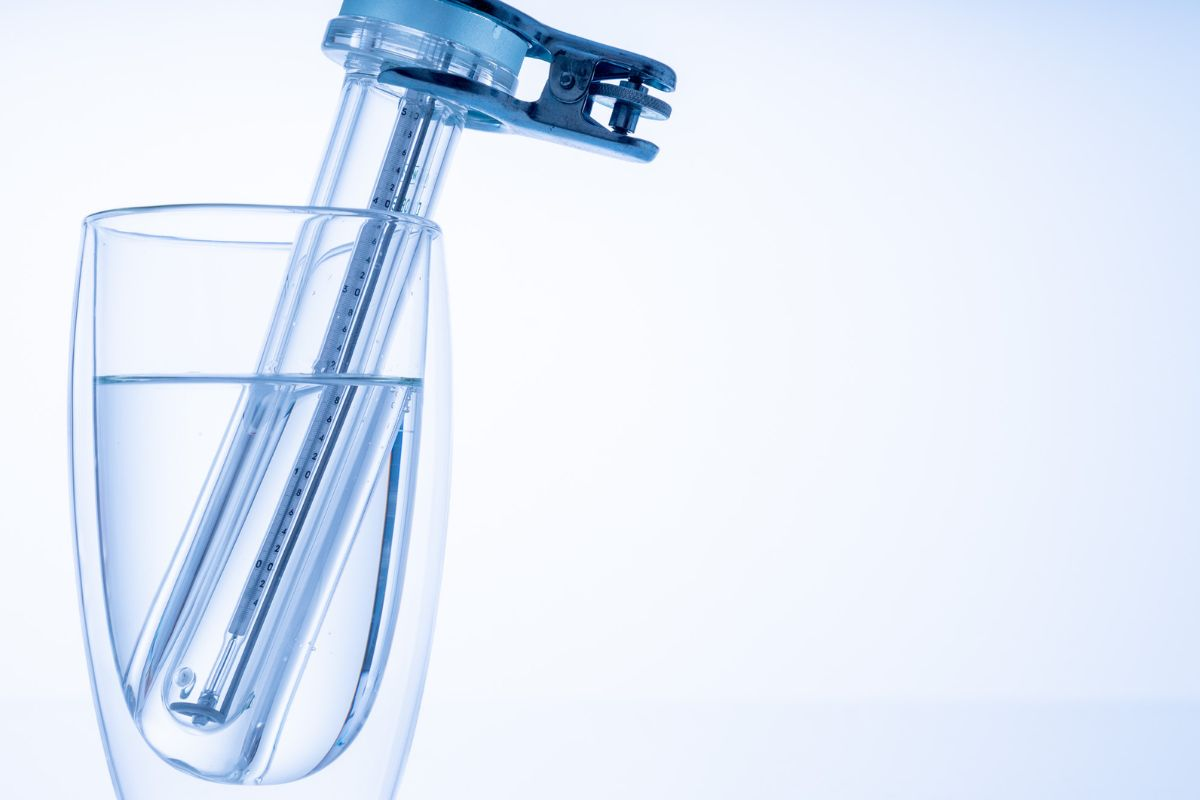# How to Find Heat Capacity of Coffee Cup Calorimeter

The amount of heat involved in a physical or chemical process is measured using a technique known as calorimetry. Heat can be described as a process of transferring energy into or from a system as a result of a temperature difference between that particular system and its surroundings. Heat is a form of energy that exists due to random motion of molecules.

Chemical reactions that involve heat transfer are done in devices known as calorimeters. A calorimeter is insulated to prevent heat gain or loss between the calorimeter and the surrounding environment. Thus, heat flow within the system can be measured more accurately. A coffee cup calorimeter is one of the most used colorimeters. In this guide, we will show you how to find heat capacity of coffee cup calorimeter.# Coffee Cup Calorimeter

A coffee cup calorimeter is ideal for measuring heat flow in chemical solutions. It is typically used in a chemical reaction with little or no change in volume. It is not used for reactions that involve gases as they can escape from the coffee cup calorimeter. Also, it is not used for reactions involving high temperatures as the cup can melt.

As a constant pressure calorimeter, the heat measured in it equals the change in enthalpy. Also, the heat is almost equal to the change occurring in the internal energy. Although its technical name is an isobaric calorimeter, it is referred to as a “coffee cup” since the experiment is done in a basic Styrofoam cup. The Styrofoam material creates an ideal adiabatic wall that keeps all the heat absorbed or released by the reaction occurring in the cup, making it possible to measure its heat capacity.

# How to Find Heat Capacity of Coffee Cup Calorimeter

The heat capacity of a substance can be described as the amount of heat needed to raise its capacity at a given quantity by 1 degree. The amount of heat released or absorbed as a result of a chemical reaction and with the pressure remaining constant is known as the enthalpy of the reaction (ΔH). In case the chemical reaction in question is an exothermic one, heat is released. Hence, the system’s temperature increases.

Essentially, the components making up a calorimeter would not absorb heat. However, the components always absorb a certain amount of energy from within the system. To measure the calorimeter’s heat capacity, you will need to do it experimentally. The simplest method is by studying a mixture of cold and hot water in a colorimeter.

In the experiment, you mix equal amounts of cold and hot water in a coffee cup calorimeter. Next, you will measure the final temperature of the equilibrium. If the calorimeter does not absorb any heat, the temperature of the equilibrium is taken to be the average of the cold and hot water temperatures.

Essentially, the hot water will lose some heat to both the calorimeter and the cold water. Therefore, the amount of heat that the cold water gains should be equivalent to the amount of heat that the hot water loses. Any difference should be the amount of heat that the calorimeter gains. In this experiment, the assumption is that there is no heat lost to the surroundings of the calorimeter.

To calculate the heat capacity of coffee cup calorimeter, use the following formula:

C = m×sp_heat

And, q = C×ΔT

Therefore, C = q ÷ ΔT

Thus, the heat capacity of coffee cup calorimeter, C = q / ΔT

Where;

C= the heat capacity of coffee cup calorimeter.

m= mass of the solution in grams.

sp_heat= the amount of heat needed to raise the temperature of a substance (1 gram) by 1 degree.

q= heat measured in joules (J).

ΔT= change in temperature.

# Summary

By now, you have learnt how to find heat capacity of coffee cup calorimeter. For this measurement, it is assumed that the heat involved in the reaction all goes into the change in water temperature. Since the measurement is done at constant pressure, the heat equals the enthalpy change. If the temperature increases, the reaction is exothermic. Also, if the temperature reduces, then the reaction is endothermic.